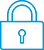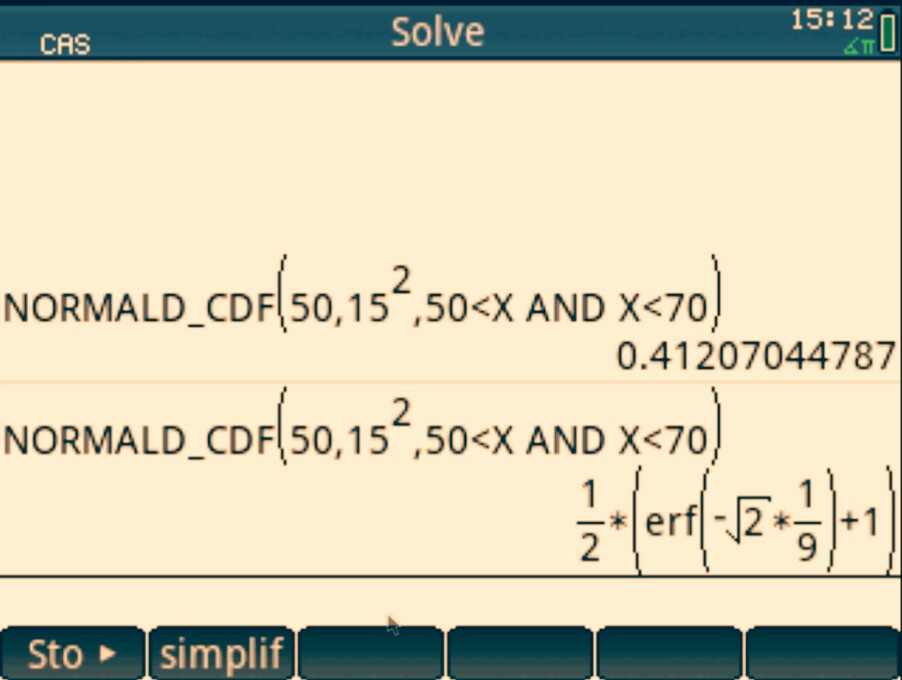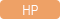cancel
Showing results for
Search instead for
Did you mean:It has been a while since anyone has replied. Simply ask a new question if you would like to start the discussion again.Level 3
45 19 1 1
Message 1 of 6
1,902
Flag Post

Solved!

# Standard Normal DistributionHP Recommended
HP PRIME

Hi,

I am trying to solve the following equation:

X - N(50,15^2)    Find Pr(50<X<70)

When I type the above into the calculator the following occurs:I don't understand why I get an 'erf' rather than a decimal answer.

Can someone please help me with using the standard normal distribution function on HP Prime calculator.

Thanks,

Arthur

Tags (1)
5 REPLIES 5Level 9
3,551 3,490 180 541
Message 2 of 6
Flag PostHP Recommended

Hi!, @Athanasios:

1) Approximate Mode.

2) Exact Mode.

erf : Returns the  floating  point value of the error function at x=a.Kind Regards !.
Have a nice day !.
@Maké (Technical Advisor Premium - HP Program Top Contributor).
Provost in HP Spanish Public Forum ... https://h30467.www3.hp.com/Level 3
45 19 1 1
Message 3 of 6
Flag PostHP Recommended

Hi Make,

Thank you very much.

Can you please direct me to an explanation of 'erf' and the concept behind exact values (on the HP Prime)?

Is there anything I can read to help me understand this mathematical concept and why certain mathematical applications require different accuracy calculator settings, so I know how to set my calculator accordingly.

Regards,

ArthurLevel 9
3,551 3,490 180 541
Message 4 of 6
Flag PostHP Recommended

Hi!, @Athanasios:

See, the description, of 'erf' by Tim Wessman, from ... http://h30434.www3.hp.com/t5/Calculators/HP-Prime-erf-function/td-p/5047983

Kind Regards !.
Have a nice day !.
@Maké (Technical Advisor Premium - HP Program Top Contributor).
Provost in HP Spanish Public Forum ... https://h30467.www3.hp.com/Level 8
747 744 121 187
Message 5 of 6
Flag PostHP Recommended

Please do not change your CAS settings as described. It generally makes more problems then helps until you understand how things are working a bit more. Default settings are nearly always going to be better for most users.

Basically, the reason this is happening is because you are in the CAS screen. Click home, try it again, and you'll see it does come out a decimal. Putting a decimal value at the end of one of your integers in the CAS screen would ALSO return a decimal result (as you are telling the CAS "please calculate this using apprixmated numerical values since I gave you an approximate numerical value with a decimal"), as would hitting the SHIFT-ENTER function to approximate the last calculation after you get that result.  Any of those are better solutions instead of changing settings like described.

As to what the erf( ) is, that is actually the way in which normal distributions are calculated! See this link for a great description: http://mathworld.wolfram.com/NormalDistributionFunction.html

Quoting from that page: "Neithernor erf can be expressed in terms of finite additions, subtractions, multiplications, and root extractions, and so must be either computed numerically or otherwise approximated."

In essence, the CAS is giving you the exact, symbolic representation of your normal distribution using a function that represents a certain operation. These types of "special functions" become more common in higher math/engineering type calculations.

When talking about exact/approximate, mathematicians are referring to the idea that something is a "perfect representation of the concept of that thing" or just a "number that is close enough to it".  For example, +infinity is a concept that the CAS actually can understand, but a normal calculator couldn't - it couldn't hold a number large enough to be "infinite". Yet in many cases, just putting in a really big number in the calculation is "good enough" to represent the idea of positive inifinity.

Another good example of this is pi. Pi itselft cannot be represented as a perfect decimal number, but in most cases a rounded off value is "close enough".   The Prime in CAS mode takes any perfect integer with no decimal to be the "idea of that number". For example SQRT(2) will return SQRT(2) in the CAS screen. There isn't a number that can simplify further down into. If you try doing SQRT(2.) (<----- note the . after the two), you are really saying "take the square root of the number that is 'close enough' to the perfect value of 2". The calculator then returns a number that is the square root "close enough" to the actual perfect, exact result.

If you do get an exact result and want to see the decimal approximation, just press SHIFT ENTER. That little squiggly equal sign is the mathematical symbol for "approximates to".

As to the last question, use this rule of thumb to help you as you get going - if you are trying to get an approximate decimal number result, the home screen is where you should be working. If you are wanting exact, symbolic algebra results, the CAS screen is where you should work. If you are in the CAS screen and want a decimal result, press shift ENTER to run the "approx" command which will do a one time evaluation of your last result to a decimal. Make sense?

TW

Although I work for the HP calculator group as a head developer of the HP Prime, the views and opinions I post here are my own.
Tags (1)Level 3
45 19 1 1
Message 6 of 6
Flag PostHP Recommended

Thank you.

† The opinions expressed above are the personal opinions of the authors, not of HP. By using this site, you accept the Terms of Use and Rules of Participation